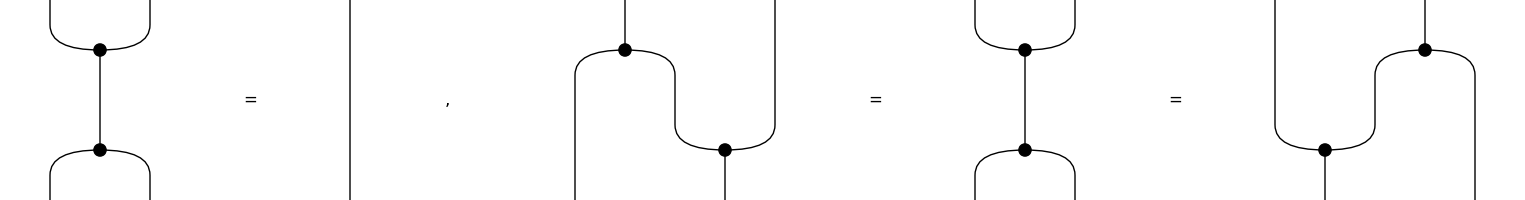# Equation#

class discopy.drawing.legacy.Equation(*terms, symbol='=', space=1)[source]#

Bases: `object`

An equation is a list of diagrams with a dedicated draw method.

Parameters:
• terms (monoidal.Diagram) – The terms of the equation.

• symbol – The symbol between the terms.

• space – The space between the terms.

Example

```>>> from discopy.tensor import Spider, Swap, Dim, Id
>>> dim = Dim(2)
>>> mu, eta = Spider(2, 1, dim), Spider(0, 1, dim)
>>> delta, upsilon = Spider(1, 2, dim), Spider(1, 0, dim)
>>> special = Equation(mu >> delta, Id(dim))
>>> frobenius = Equation(
...     delta @ Id(dim) >> Id(dim) @ mu,
...     mu >> delta,
...     Id(dim) @ delta >> mu @ Id(dim))
>>> Equation(special, frobenius, symbol=', ').draw(
...          aspect='equal', draw_type_labels=False, figsize=(8, 2),
...          path='docs/_static/drawing/frobenius-axioms.png')
```draw(path=None, space=None, **params)[source]#

Drawing an equation.

Parameters: